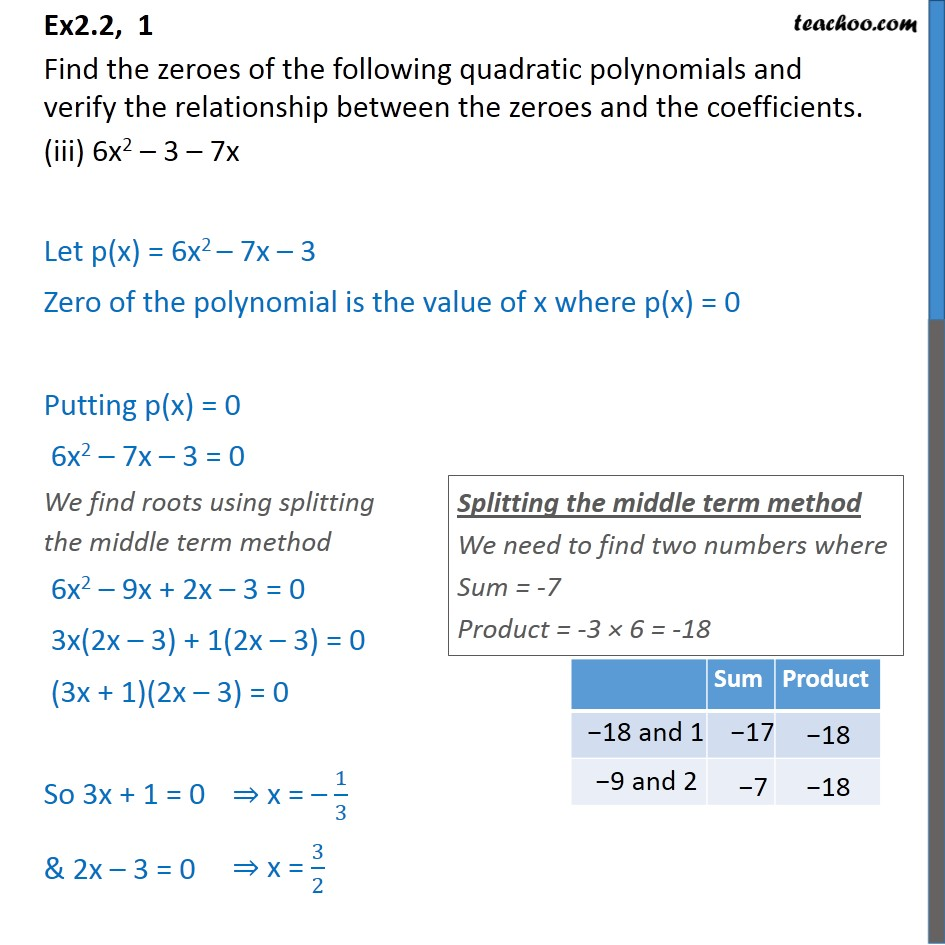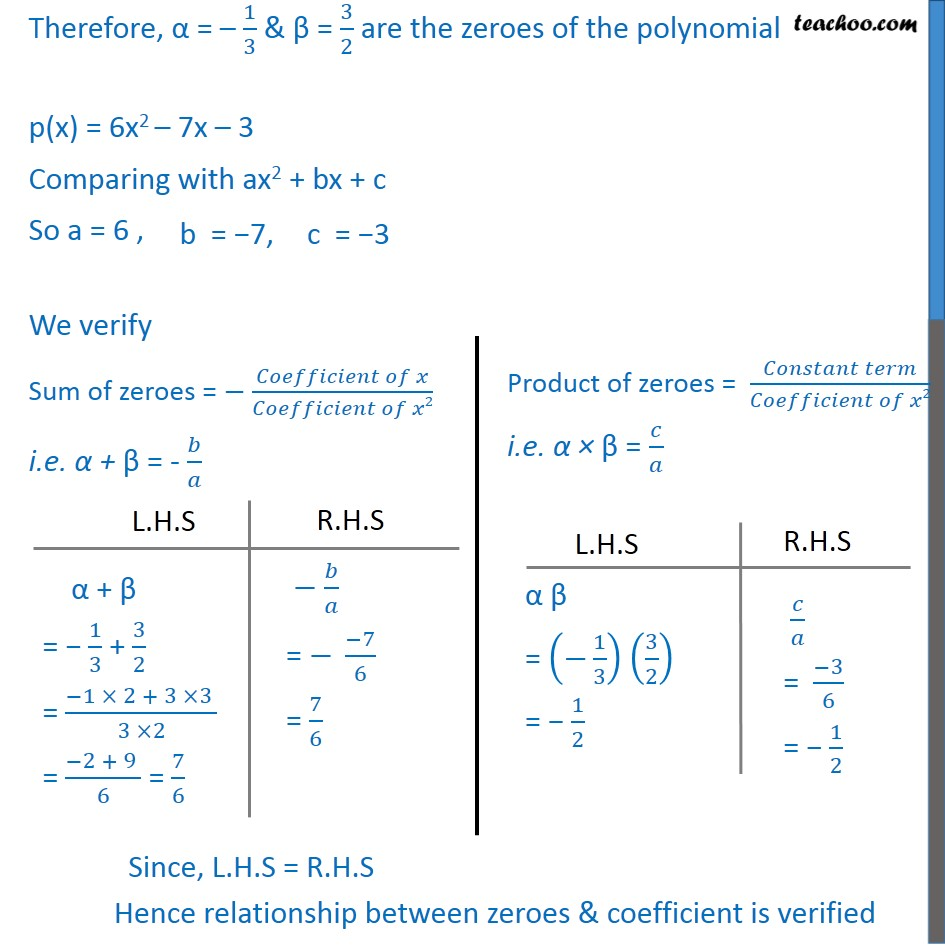1. Chapter 2 Class 10 Polynomials (Term 1)
2. Serial order wise
3. Ex 2.2

Transcript

Ex2.2, 1 Find the zeroes of the following quadratic polynomials and verify the relationship between the zeroes and the coefficients. (iii) 6x2 – 3 – 7x Let p(x) = 6x2 – 7x – 3 Zero of the polynomial is the value of x where p(x) = 0 Putting p(x) = 0 6x2 – 7x – 3 = 0 We find roots using splitting the middle term method 6x2 – 9x + 2x – 3 = 0 3x(2x – 3) + 1(2x – 3) = 0 (3x + 1)(2x – 3) = 0 So 3x + 1 = 0 & 2x – 3 = 0 Therefore, α = – 1/3 & β = 3/2 are the zeroes of the polynomial p(x) = 6x2 – 7x – 3 Comparing with ax2 + bx + c So a = 6 , We verify Sum of zeroes = − (𝐶𝑜𝑒𝑓𝑓𝑖𝑐𝑖𝑒𝑛𝑡 𝑜𝑓 𝑥)/(𝐶𝑜𝑒𝑓𝑓𝑖𝑐𝑖𝑒𝑛𝑡 𝑜𝑓 𝑥2) i.e. α + β = - 𝑏/𝑎